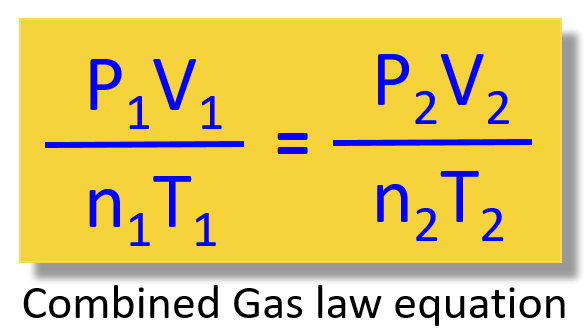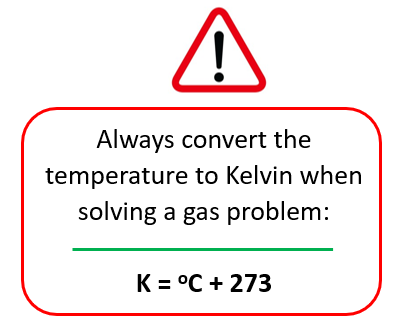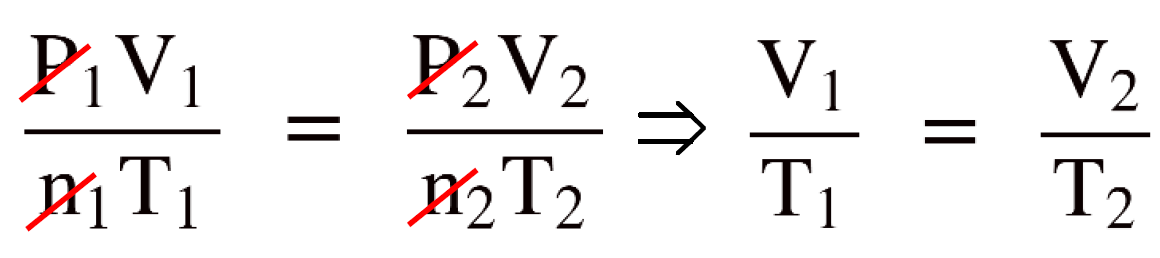## General Chemistry

How do you know which ideal gas equation to use when solving a problem?

Fortunately, there is a short answer to this, and it is the combined gas law equation:It allows you not to memorize all the other gas laws and decide every time which one to use when solving a problem.

For example,

What will be the final volume of a 3.50 L sample of nitrogen at 20.0 °C if it is heated to 200. °C?

First, write down what is given and what needs to be determined:

V1 = 3.50 L
T1 = 20 oC
T2 = 200. oC
V2 = ?

Second, remember to always convert the temperature to Kelvin in any gas problem:So, T1 = 20 + 273 = 293 K,  T2 = 200 + 273 = 473 K

We solved this problem last time when talking about the ideal gas laws, however, there we needed to remember or figure out that it is based on Charle’s law.

Suppose we don’t know which law to use. For this what you do is:

Write the combined gas law equation and cancel the constants. In this case, n and P because there is nothing mentioned about them.By canceling the constants in the combined gas law, we now have the Charle’s law and to determine V2 , we just need to rearrange the equation and plug the numbers:

$\frac{{{{\rm{V}}_{\rm{1}}}}}{{{{\rm{T}}_{\rm{1}}}}}\; = \;\frac{{{{\rm{V}}_{\rm{2}}}}}{{{{\rm{T}}_{\rm{2}}}}}$

${{\rm{V}}_{\rm{2}}}\; = \;\frac{{{\rm{473}}\;\cancel{{\rm{K}}}\;{\rm{ \times }}\;{\rm{3}}{\rm{.50}}\;{\rm{L}}}}{{{\rm{293}}\;\cancel{{\rm{K}}}}}\;{\rm{ = }}\;{\rm{5}}{\rm{.65}}\;{\rm{L}}$

Let’s do one more example using the combined gas law equation when three parameters are being changed.

A gas sample is stored in a 429 mL container at 9.50°C and 2.20 atm. Calculate the pressure of the gas if the volume changes to 134 mL and the container heated to 134.5°C? Assume a constant amount of gas.

First assign the states and write down what is given and what needs to be determined:

P1 = 2.20 atm
T1 = 9.50°C
V1 = 429 mL
T2 = 134.5°C
V2 = 134 mL
P2 = ?

Next, write the combined gas law equation, and remove the moles (n) because there is nothing mentioned about them, and we assume that they do not change:

$\frac{{{{\rm{P}}_{\rm{1}}}{{\rm{V}}_{\rm{1}}}}}{{{{\rm{T}}_{\rm{1}}}}}\; = \;\frac{{{{\rm{P}}_{\rm{2}}}{{\rm{V}}_{\rm{2}}}}}{{{{\rm{T}}_{\rm{2}}}}}$

${{\rm{P}}_{\rm{2}}}\; = \;\frac{{{{\rm{P}}_{\rm{1}}}{{\rm{V}}_{\rm{1}}}{{\rm{T}}_{\rm{2}}}}}{{{{\rm{T}}_{\rm{1}}}{{\rm{V}}_{\rm{2}}}}}$

Make sure the temperature are in Kelvin:

T1 = 9.50 + 273 = 282.5 K

T2 = 134.5° + 273 = 407.5 K

Finally, enter the numbers to determine P2:

${{\rm{P}}_{\rm{2}}}\; = \;\frac{{{{\rm{P}}_{\rm{1}}}{{\rm{V}}_{\rm{1}}}{{\rm{T}}_{\rm{2}}}}}{{{{\rm{T}}_{\rm{1}}}{{\rm{V}}_{\rm{2}}}}}\; = \;\frac{{{\rm{2}}{\rm{.20}}\;{\rm{atm}}\;{\rm{ \times }}\;{\rm{429}}\;\cancel{{{\rm{mL}}}}\;{\rm{ \times }}\;{\rm{407}}{\rm{.5}}\;\cancel{{\rm{K}}}}}{{{\rm{282}}{\rm{.5}}\;\cancel{{{\rm{K}}\;}}\;{\rm{134}}\;\cancel{{{\rm{mL}}}}}}\;{\rm{ = }}\;{\rm{10}}{\rm{.2}}\;{\rm{atm}}$

# When to Use the Ideal Gas Law Equation?

This strategy can be used to solve any gas problem when the P, V, N, and T parameters are changing. If it is a stationary system and you are asked to determine the n, P, V, or T, then you need to use the ideal gas law equation: PV = nRT

For example,

What is the pressure in a 26.0 L container with 5.40 moles of nitrogen dioxide if the temperature is 64.0 °C?

First, write down what is given and what needs to be detemined:

V = 26.0 L
n (NO2) = 5.40 mol
T = 64.0 oC
P = ?

Convert the temperature to Kelvin:

T = 273 + 64.0 = 337 K

To find the pressure, we need the ideal gas law equation:

PV = nRT

${\rm{P}}\;{\rm{ = }}\;\frac{{{\rm{nRT}}}}{{\rm{V}}}$

${\rm{P}}\;{\rm{ = }}\;\frac{{{\rm{5}}{\rm{.40}}\;\cancel{{{\rm{mol}}}}\;{\rm{ \times }}\;{\rm{0}}{\rm{.08206}}\;\cancel{{\rm{L}}}\;{\rm{atm}}\;\cancel{{{{\rm{K}}^{{\rm{ – 1}}}}}}\;\cancel{{{\rm{mo}}{{\rm{l}}^{{\rm{ – 1}}}}}}\;{\rm{ \times }}\;{\rm{337}}\;{\rm{K}}}}{{{\rm{26}}{\rm{.0 }}\cancel{{\rm{L}}}}}\;{\rm{ = }}\;{\rm{5}}{\rm{.74}}\;{\rm{atm}}$

Check Also

1685271924